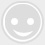# 单片机C语言之串口通信协议

-回复 -浏览（1）、物理上的连线至少3根，分别是Tx数据发送线，Rx数据接收线，GND共用地线。
（2）、0与1的约定。RS232电平，约定﹣5V至﹣25V之间的电压信号为1，﹢5V至﹢25V之间的电压信号为0 。TTL电平，约定5V的电压信号为1，0V电压信号为0 。CMOS电平，约定3.3V的电压信号为1，0V电压信号为0 。其中，CMOS电平一般用于ARM芯片中。（3）、发送秩序。低位先发。
（4）、波特率。收发双方共同约定的一个数据位（0或1）在数据传输线上维持的时间。也可理解为每秒可以传输的位数。常用的波特率有300bit/s, 600bit/s, 2400bit/s, 4800bit/s, 9600bit/s。
（5）、通信的起始信号。发送方在没有发送数据时，应该将Tx置1 。 当需发送时，先将Tx置0，并且保持1位的时间。接受方不断地侦测Rx，如果发现Rx常时间变高后，突然被拉低（置为0），则视为发送方将要发送数据，迅速启动自己的定时器，从而保证了收发双方定时器同步定时。

（6）、停止信号。发送方发送完最后一个有效位时，必须再将Tx保持1位的时间，即为停止位。

[cpp] view plain copy

1. #include "reg51.h"

2. /*

3.   将P1.0虚拟成串口发送脚TX

4.   以9600bit/s的比特率向外发送数据

5.   因为波特率是    9600bit/s

6.   所以me发送一位的时间是 t=1000000us/9600=104us

7. */

8. sbit TX=P3^1; //P1^0 output TTL signal, need to transferred to rs232 signal, can be connected to P3^1

9. #define u16 unsigned int //宏定义

10. #define u8 unsigned char

11. u8 sbuf;

12. bit ti=0;

13. void delay(u16 x)

14. {

15.     while(x--);

16. }

17. void Timer0_Init()

18. {

19.     TMOD |= 0x01;

20.     TH0=65440/256;

21.     TH0=65440%256;

22.     TR0=0;

23. }

24. void Isr_Init()

25. {

26.     EA=1;

27.     ET0=1;

28. }

29. void Send_Byte(u8 dat)

30. {

31.     sbuf=dat;//通过引入全局变量sbuf，可以保存形参dat

32.     TX=0; //A 起始位

33.     TR0=1;

34.     while(ti==0);    //等待发送完成

35.     ti=0; //清除发送完成标志

36. }

37. void TF0_isr() interrupt 1     //每104us进入一次中断

38. {

39.     static u8 i; //记录进入中断的次数

40.     TH0=65440/256;

41.     TL0=65440%256;

42.     i++;

43.     if(i>=1 && i<=8)

44.     {

45.         if((sbuf&(1<<(i-1)))==0)  // (sbuf&(1<<(i-1)))表示取出i-1位

46.         {

47.             TX=0;

48.         }

49.         else

50.         {

51.             TX=1;

52.         }

53.     }

54.     if(i==9)  //停止位

55.     {

56.         TX=1;

57.     }

58.     if(i==10)

59.     {

60.         TR0=0;

61.         i=0;

62.         ti=1; //发送完成

63.     }

64. }

65. void main()

66. {

67.     TX=1; //使TX处于空闲状态

68.     Timer0_Init();

69.     Isr_Init();

70.     while(1)

71.     {

72.         Send_Byte(65); //0x41

73.         delay(60000);

74.     }

75. }

x＝256－f/(384*b)

[cpp] view plain copy

1. #include "reg51.h"

2. #define u16 unsigned int

3. #define u8 unsigned char

4. void delay(u16 x)

5. {

6.     while(x--);

7. }

8. void Uart_Init() //串口初始化

9. {

10.     SCON=0x50; //8位异步模式

11.     TMOD|=0x20; //定时器1工作方式2

12.     TH1=253;//9600bit/s

13.     TR1=1;

14. }

15. void Send_Byte(u8 dat)

16. {

17.     SBUF=dat; //启动发送，只需要把发送内容给SBUF这个寄存器

18.     while(TI==0); //等待发送完成，因为TI为1时表示在发送停止位

19.     TI=0;

20. }

21. void main()

22. {

23.     Uart_Init();

24.     while(1)

25.     {

26.        Send_Byte('m');

27.        delay(60000);

28.     }

29. }

[cpp] view plain copy

1. #include "reg51.h"

2. #define u16 unsigned int

3. #define u8 unsigned char

4. void delay(u16 x)

5. {

6.     while(x--);

7. }

8. void Uart_Init() //串口初始化

9. {

10.     SCON=0x50; //8位异步模式

11.     TMOD|=0x20; //定时器1工作方式2

12.     TH1=253;//9600bit/s

13.     TR1=1;

14. }

15. void Send_Byte(u8 dat)    //串口发送一个字节

16. {

17.     SBUF=dat; //启动发送，只需要把发送内容给SBUF这个寄存器

18.     while(TI==0); //等待发送完成，因为TI为1时表示在发送停止位

19.     TI=0;

20. }

21.  void Send_String(u8 *str)   //发送一个字符串  *str为字符串第一个字符的地址

22.  {

23.     abc:      //标号

24.     if(*str != 0)

25.     {

26.         Send_Byte(*str);

27.         str++;

28.         goto abc;

29.     }

30.  }

31. void main()

32. {

33.     Uart_Init();

34.     while(1)

35.     {

36.        Send_String("Hello World! 第一！");

37.        Send_Byte(10);

38.        delay(60000);

39.        delay(60000);

40.     }

41. }

51单片机学习网

ID：gh_29176fc67325

▲长按二维码“识别”关注

ID：gh_e45926de778f

▲长按二维码“识别”关注